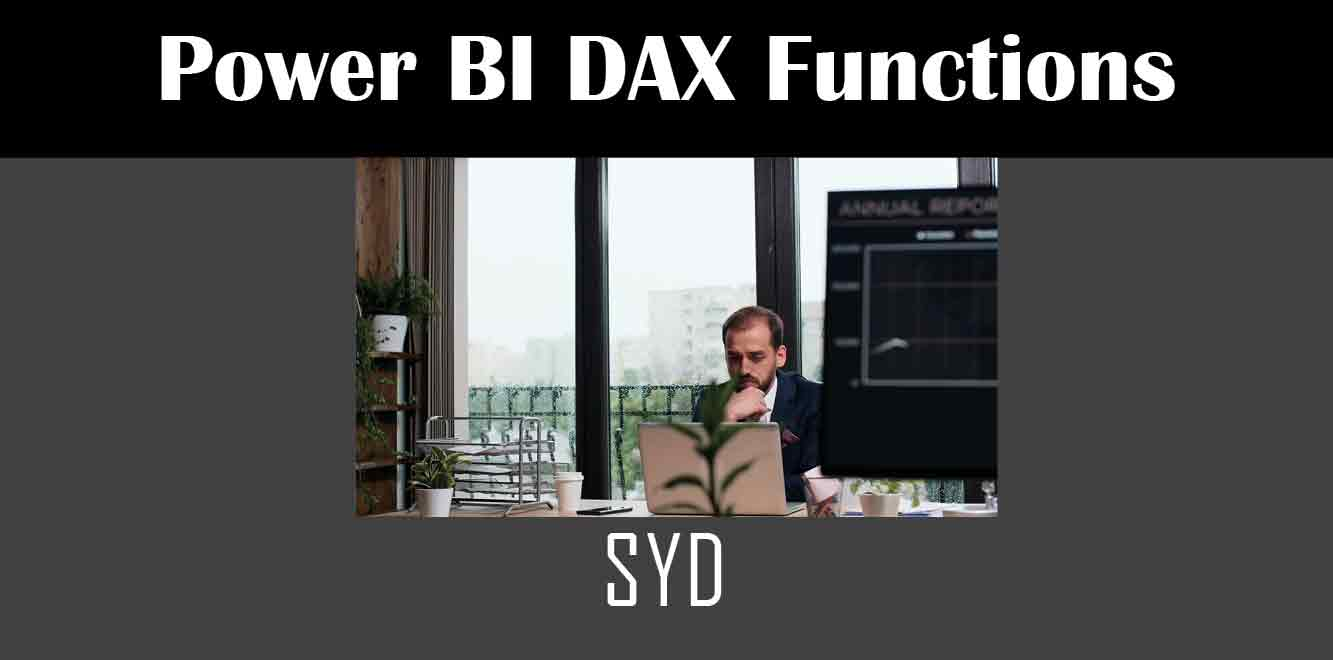# SYD

## Y## What is the SYD Function?

The SYD function is a depreciation expense calculation method that is based on the sum of the digits of an asset’s useful life. It assumes that an asset’s depreciation expense is a function of the sum of the years of the asset’s useful life. The depreciation expense is allocated throughout the asset’s useful life, with more depreciation being allocated to the early years of the asset’s life, and less depreciation being allocated to the later years.

## How to Use the SYD Function in Power BI

The SYD function in Power BI is easy to use and requires only a few inputs. The inputs required are:

– Cost: The initial cost of the asset

– Salvage: The salvage value of the asset

– Life: The useful life of the asset

– Period: The period for which you want to calculate depreciation

The formula to calculate depreciation using the SYD function is as follows:

SYD (cost, salvage, life, period)

``` Example Let's take an example to illustrate how to use the SYD function in Power BI. Suppose we have a computer that costs \$2,000, has a useful life of 5 years, and has a salvage value of \$500. We want to calculate the depreciation expense for the first year. The formula to calculate depreciation using the SYD function is as follows: ```

SYD(2000, 500, 5, 1)

``` The resulting depreciation expense for the first year will be \$800. Advantages of Using the SYD Function There are several advantages to using the SYD function for depreciation calculations: 1. Saves time: The SYD function can save time, especially if you need to calculate depreciation for multiple assets. 2. Easy to use: The SYD function is easy to use and requires only a few inputs. 3. Accurate: The SYD function is an accurate method for calculating depreciation expenses. 4. Flexible: The SYD function is a flexible method for calculating depreciation, as it allows for more depreciation to be allocated to the early years of an asset's life. Limitations of Using the SYD Function There are also some limitations to using the SYD function: 1. Assumes even depreciation: The SYD function assumes that depreciation is allocated evenly throughout the useful life of the asset, which may not be the case for all assets. 2. Requires accurate inputs: The SYD function requires accurate inputs, such as the initial cost, the salvage value, and the useful life of the asset. 3. Limited to straight-line depreciation: The SYD function is limited to straight-line depreciation, which may not be appropriate for all assets. The SYD function in Power BI is a powerful tool for calculating depreciation expenses for assets. It is easy to use and requires only a few inputs. The SYD function is an accurate method for calculating depreciation expenses and is flexible in allocating more depreciation to the early years of an asset's life. However, it is limited to straight-line depreciation and assumes even depreciation, which may not be appropriate for all assets. Overall, the SYD function is a useful tool for financial analysis in Power BI. Power BI DAX Training Courses by G Com Solutions (0800 998 9248)Power BI DAX Intensive Training Course £1,050.00 – £26,550.00 Select optionsContinue Loading DonePower BI DAX Introduction £395.00 – £9,750.00 Select optionsContinue Loading DonePower BI DAX Intermediate £395.00 – £9,750.00 Select optionsContinue Loading DonePower BI DAX Advanced £395.00 – £9,750.00 Select optionsContinue Loading Done Upcoming Courses Contact Us Subject Your Name (required) Company/Organisation Email (required) Telephone Training Course(s) Power BI Intensive TrainingPower BI introduction Power BI IntermediatePower BI AdvancedDAXPower Query MPower BI CertificationPower BI AdministrationPower PlatformPower AutomatePower AppsOTHER Your Message Upload Example Document(s) (Zip multiple files) ```
``` ```
``` ```
``` ```
``` ```
``` ```